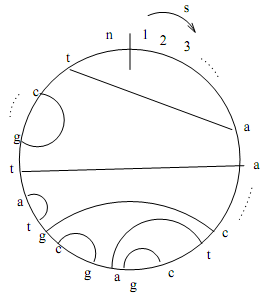### Compute a shortest superstring

Assignment Help Theory of Computation
##### Reference no: EM13768

1.

Let s1 and s2 be two strings of lengths m and n respectively. By de?nition, a superstring of s1 and s2 is one which contains s1 and s2 as substrings. Give a dynamic programming algorithm to compute a shortest superstring of two strings, and analyze its complexity.

Example: If s1 = aaaagatacac and s2 = acacggtttt then the following are all valid superstrings:
{aaaagatacacggtttt, aaaagatacacacggtttt, aaaagatacacacacggtttt}

However, aaaagatacacggtttt is the shortest. Note that the above formulation does not allow any variations (mismatches or gaps) within the overlapping region.

The motivating application for this problem is genome assembly, where the goal is to reconstruct an unknown supersequence by assembling smaller (known) fragments obtained from it.

2. Give a dynamic programming algorithm for the problem of ?nding an optimal local alignment between a string and itself - ie., we wish to ?nd a pair of best aligning substrings within s.

Note that we cannot directly use the Smith-Waterman local alignment algorithm because it will then only output the entire string aligned to itself as its ?nal answer, which is not the answer we want here. We are after a pair of shorter substrings within s. To make this routine more biologically relevant, you can assume that any optimally aligning pair of substrings that your algorithm detects should NOT overlap within the string - i.e., your optimal local alignment should correspond to two substrings s[i1 . . . i2] and s[j1 . . . j2], such that i2 < j1 (without loss of generality).

The motivating application for this problem is to ?nd "genomic repeats", which are repetitive substrings (with a few variations) present in different loci along a long genome.

3.

How will you modify the linear space Hirschberg technique to work for optimal local alignment computation in linear space (both opt. local alignment score & traceback path)? Explain the main steps of your new (modi?ed) algorithm. No need to expand on parts that are identical to the version of the algorithm discussed for global alignment computation in class.

4.

A q-gram1 is de?ned as a string of length q, where q > 0 is a "small" constant (say, q < 10). Given a string s, let Qq s denote the set of all q-grams in s. Given two strings s1 and s2 of lengths m and n respectively, their q-gram distance is the sum of the number of unique q-grams in each - i.e., qd(s1, s2) = |Qq s1\Qq s2 | + |Qq s2\Qq s1

(Note: the pipe symbol '|' in this expression stands for set cardinality - not absolute values; also the \ symbol stands for set difference.)

a) Give an algorithm to compute qd(s1, s2).

b) Derive a tight lower-bound for edit distance between two strings as a function of their q-gram distance.

c) Using the above results, argue how we can save time in practice in the following scenario: we are interesting in knowing the (optimal) edit distance between two strings only if it is below a certain cutoff, say τ . Otherwise, we don't care what is output.

5.

Given a pattern P of length m and a text T of length n, give an algorithm to ?nd and report all occurrences of P in T using the look-up table data-structure. You can assume that n > m > k, where k is the window length used to build the lookup table.

6.

The nested pairing problem:

Let s be a DNA sequence of length n, as illustrated in Figure 1. We de?ne "pairing" as a set of disjoint pairs of indices in s. A pairing is "proper" only if it is one of the following four pairs: {(a, t), (t, a), (c, g), (g, c)}. Any proper pairing becomes a "nested pairing" when the
string index intervals covered by no two pairs overlap. Put another way with reference to the Figure 1, no two edges should criss-cross.
The problem for this question is to ?nd an optimal nested pairing - i.e., a nested pairing with maximum cardinality.

Give an e?cient algorithm to compute an optimal nested pairing using dynamic programming and comment on its runtime and space requirements.

PS: As a reference, my solution for this problem takes O(n3) time.Figure: Illustration of a nested pairing of a string s of length n. Each pair is shown as an edge connecting those two character locations along the string. Note that the sequence shown s is *not* a circular string, as the start index (1) and end index (n) are clearly marked. It is only shown as circular for ease of illustrating the nested pairing.

### Previous Q& A

#### Cost-benefit analysis

Create a cost-benefit analysis to evaluate the project

#### Determine the temperature of the electric heater element

Determine the temperature of the electric heater element and drawing of the temperature profile.

#### Determine the heat transfer characteristics

To determine the heat transfer characteristics of a cylinder under cross flow conditions when the cylinder is isolated.

#### Economic integration

Economic integration is the establishment of transnational rules and regulations that enhance economic trade and cooperation among countries.

#### Data structures for a single algorithm

Data structures for a single algorithm

#### Calculate the expected value of returns of stock

Calculate the expected value of returns of stock.

#### Development of human resource management system

Development of Human Resource Management System for the Health Service.

#### In praise of price gouging

Using two graphs, show consumer surplus before and after government intervention.

#### Write paper on inventory management system

Write paper on Inventory Management System.

### Similar Q& A

#### Redundant sequence identi cation

Redundant sequence identi cation

#### Finite-state machine design

Create a finite-state machine design to turn your FPGA development board into a simple programmable music box.